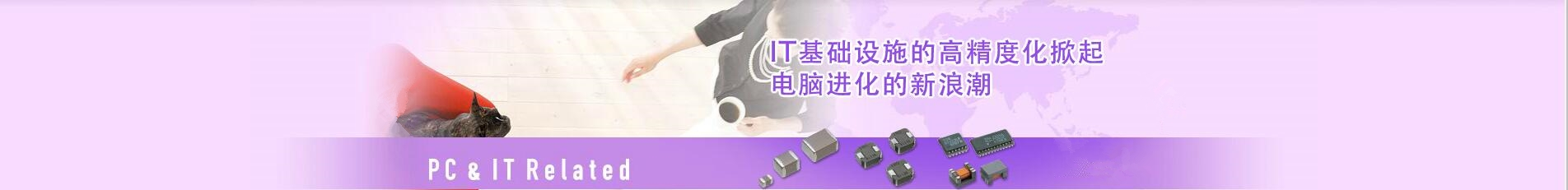## 日本TDK村田murata一级代理商-提供贴片电容器_电感器_蜂鸣器_滤波器_电阻磁珠_电源等产品代理服务

### 深圳市智成电子有限公司

Shenzhen Zhicheng Electronic Co., Ltd.

## 0755-23282269# 高精密电阻阻值表_高精密电阻阻值对照表

A=10^0=1

B=10^1=10

C=10^2=100

D=10^3=1000

E=10^4=10000

F=10^5=100000

X=R=10^（-1）=1/10

Y=S=10^（-2）=1/100

24x:17.4（欧姆）

24B:1.74k（欧姆）

24C:17.4k（欧姆）